# RD Sharma Solutions Class 9 Maths Chapter 4 Algebraic Identities Exercise 4.3

RD Sharma Class 9 Maths Chapter 4 exercise 4.3 available here. This exercise is about identity for the cube of a binomial. In this exercise, we shall extend the identity for the square of a binomial to the cube of a binomial. To score good marks in the examination, students are advised to solve all the questions enlisted in RD Sharma Class 9. Students can download Class 9 Maths Chapter 4 PDF from below link.

## Download PDF of RD Sharma Solutions for Class 9 Maths Chapter 4 Algebraic Identities Exercise 4.3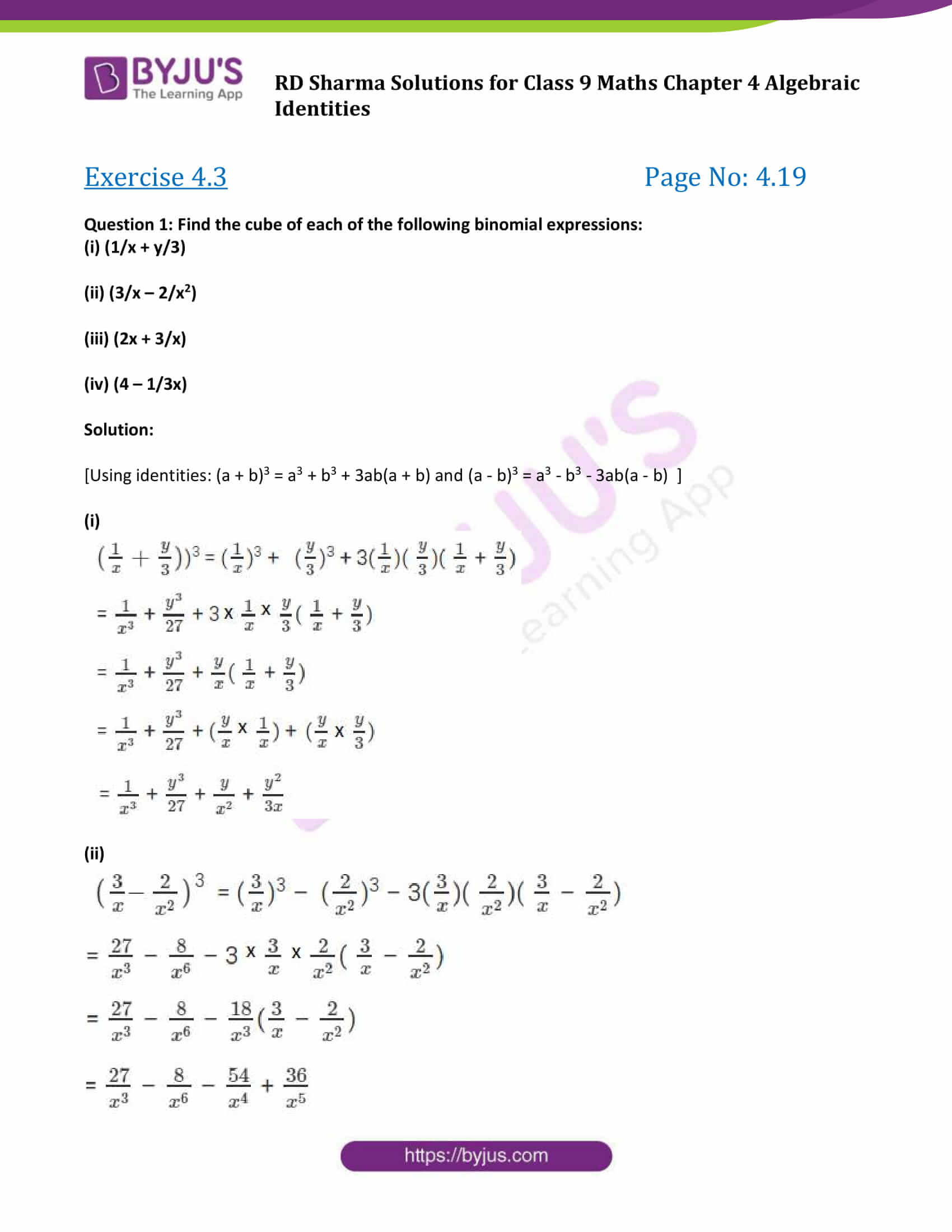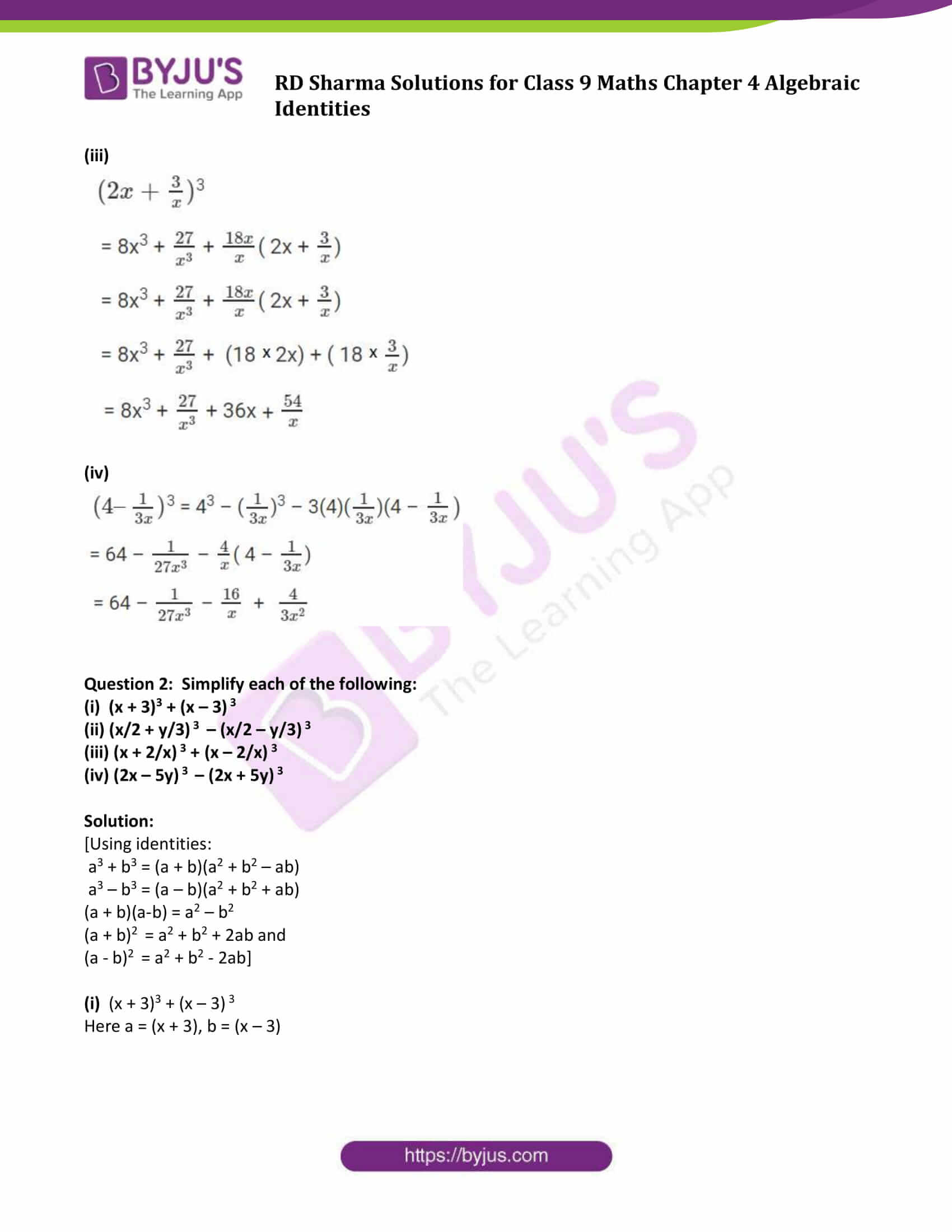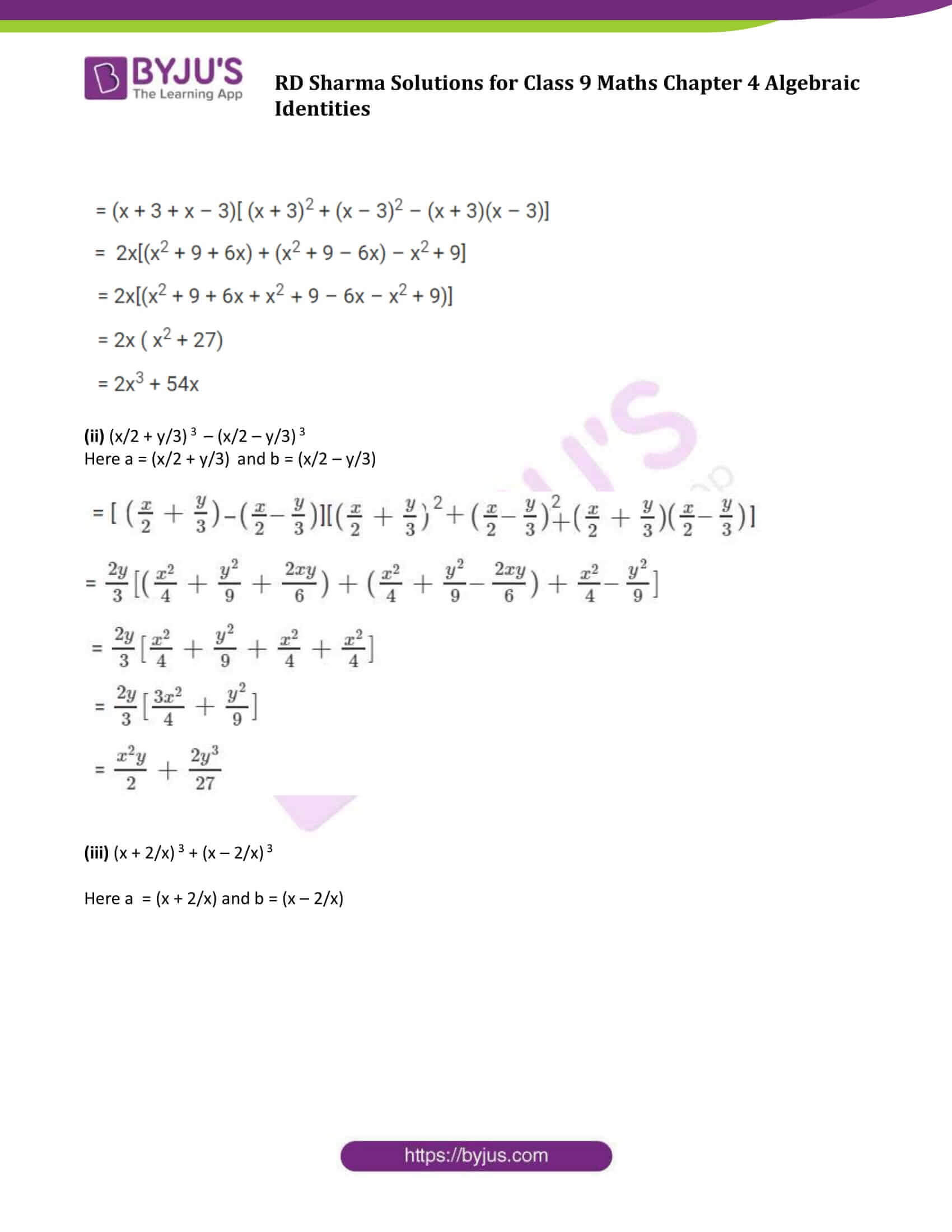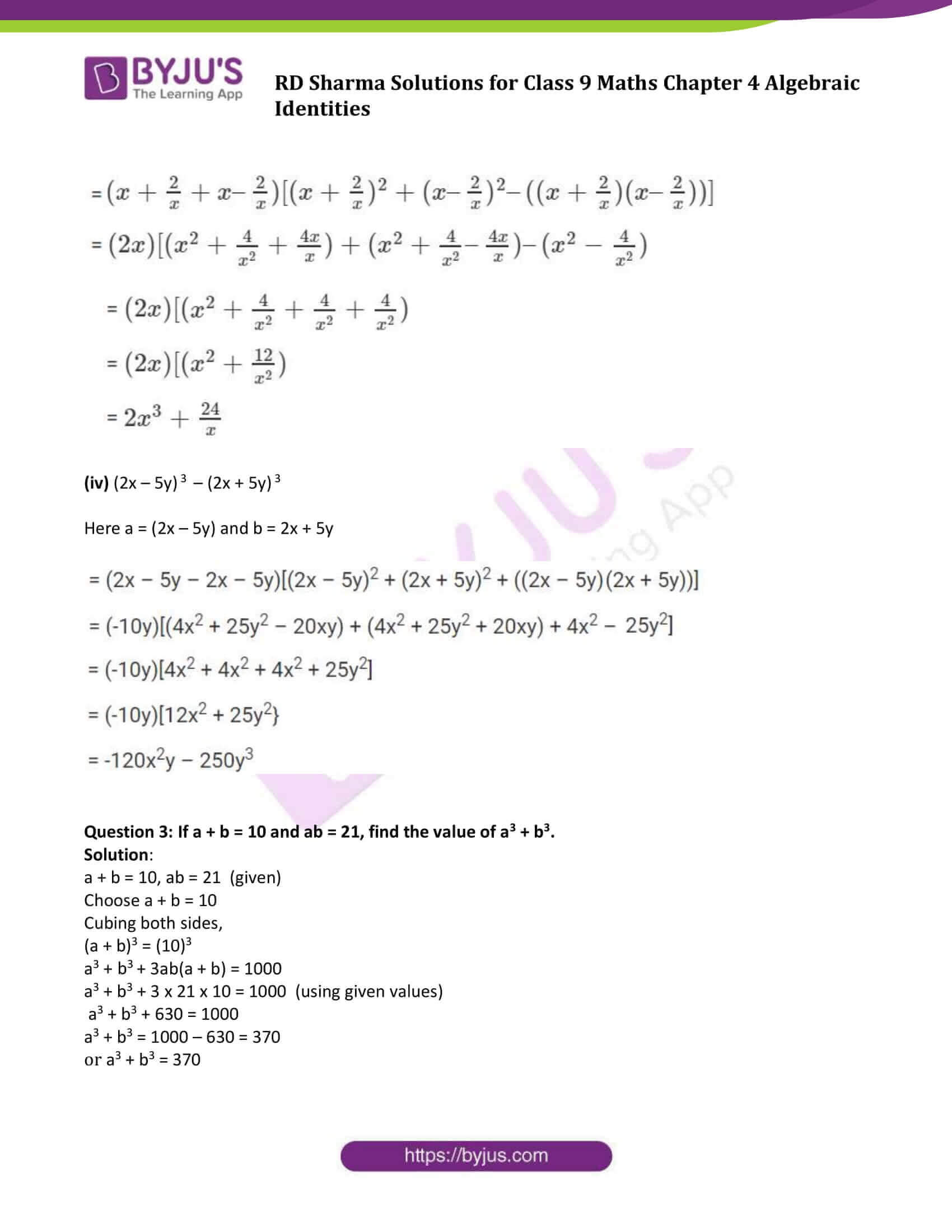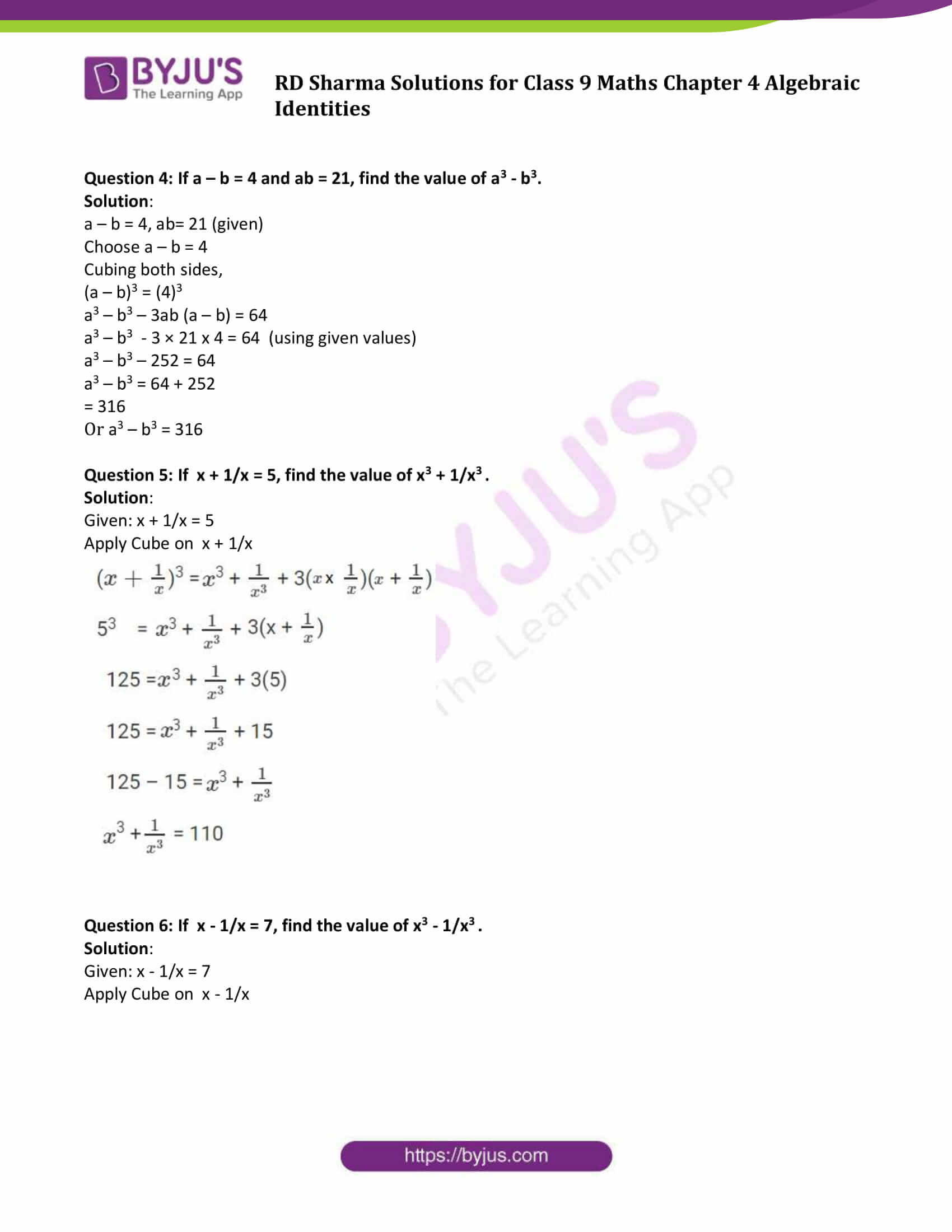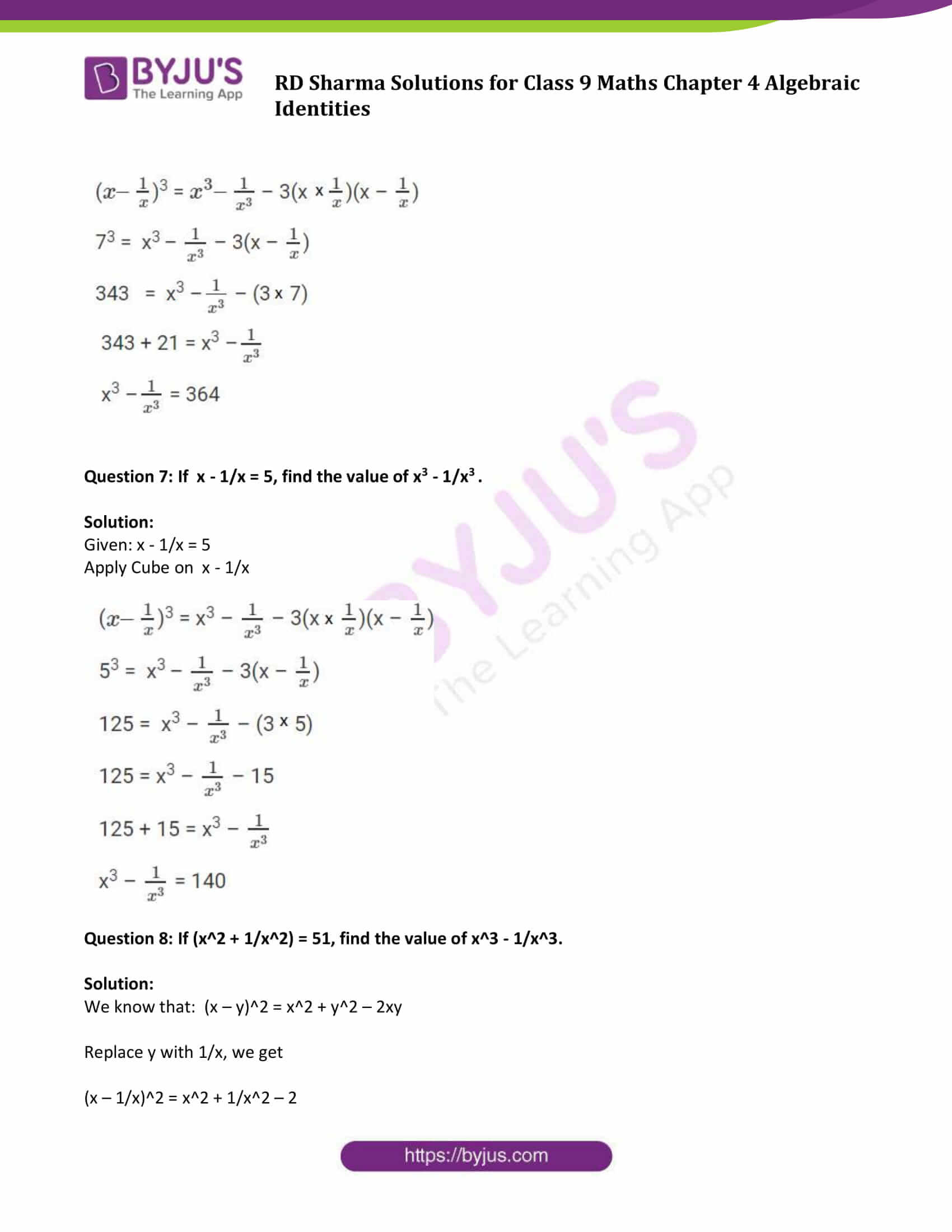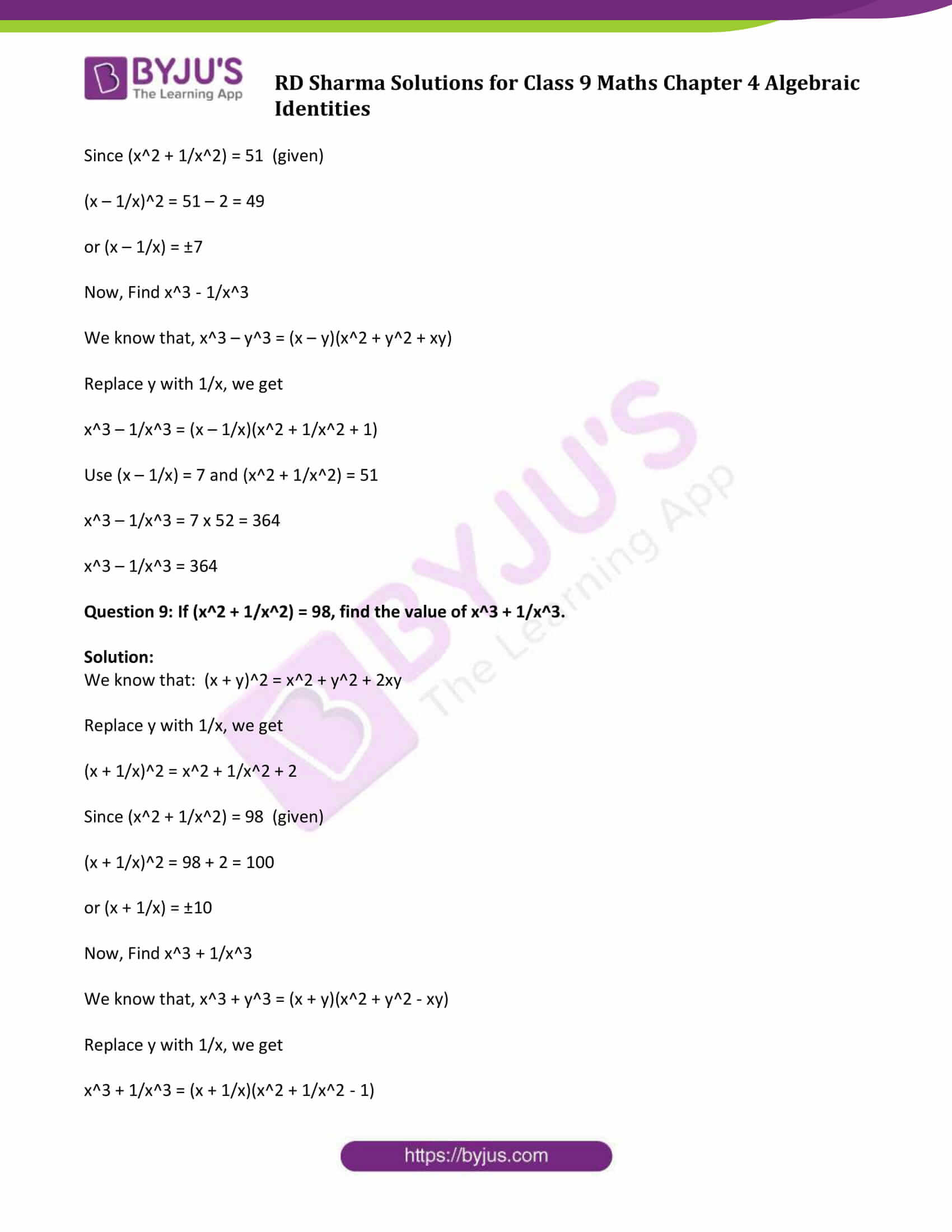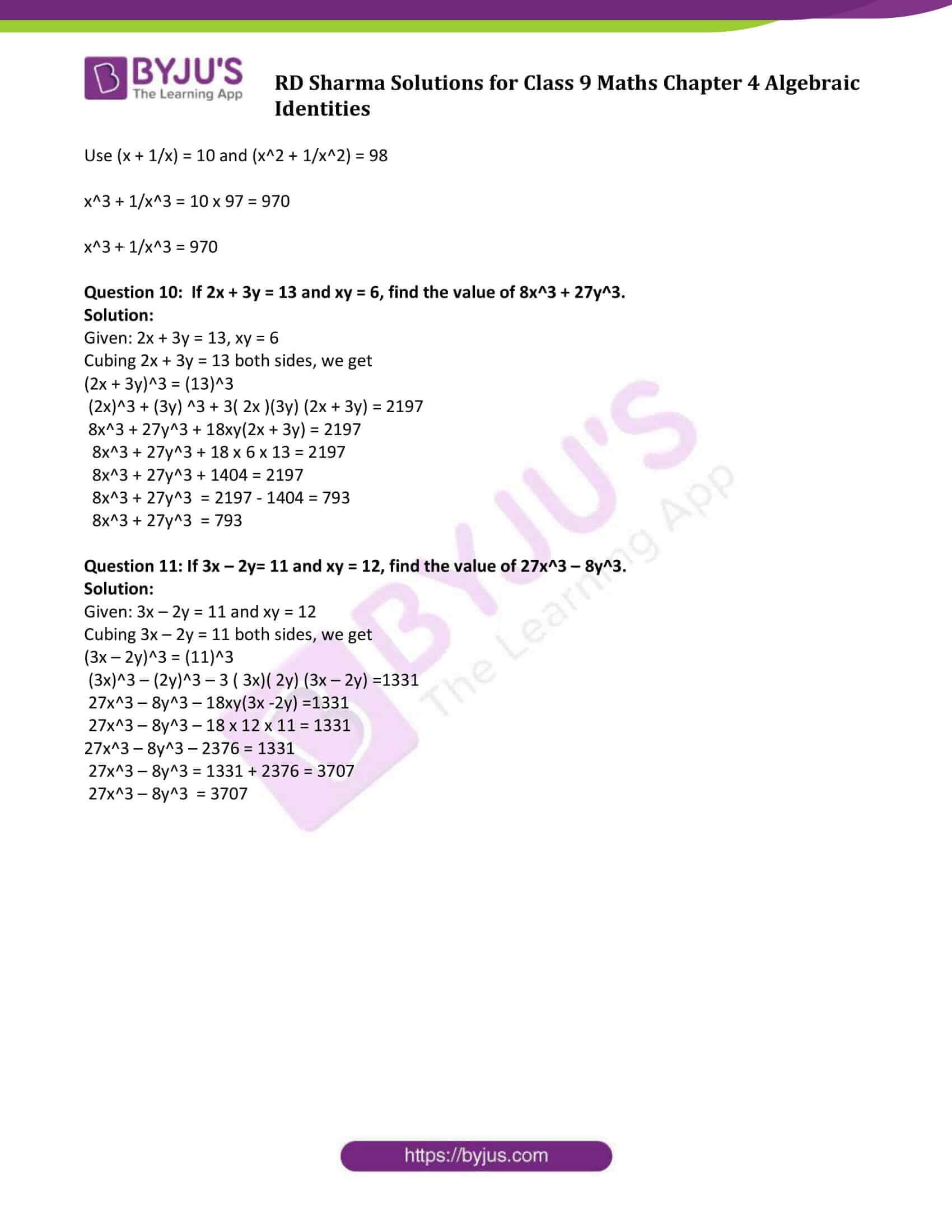### Access Answers to Maths RD Sharma Solutions for Class 9 Chapter 4 Algebraic Identities Exercise 4.3 Page number 4.19

#### Exercise 4.3 Page No: 4.19

Question 1: Find the cube of each of the following binomial expressions:

(i) (1/x + y/3)

(ii) (3/x – 2/x2)

(iii) (2x + 3/x)

(iv) (4 – 1/3x)

Solution:

[Using identities: (a + b)3 = a3 + b3 + 3ab(a + b) and (a – b)3 = a3 – b3 – 3ab(a – b) ]

(i)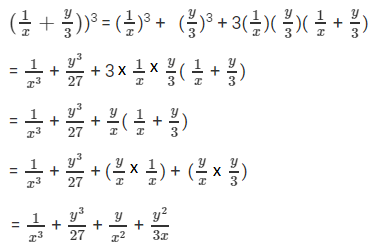(ii)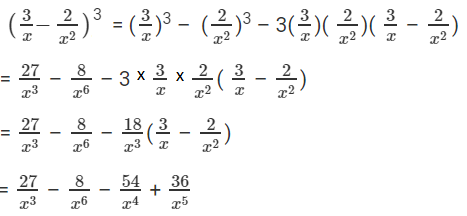(iii)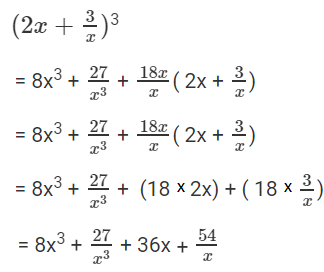(iv)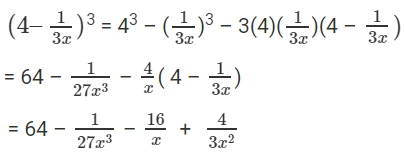Question 2: Simplify each of the following:

(i) (x + 3)3 + (x – 3) 3

(ii) (x/2 + y/3) 3 – (x/2 – y/3) 3

(iii) (x + 2/x) 3 + (x – 2/x) 3

(iv) (2x – 5y) 3 – (2x + 5y) 3

Solution:

[Using identities:

a3 + b3 = (a + b)(a2 + b2 – ab)

a3 – b3 = (a – b)(a2 + b2 + ab)

(a + b)(a-b) = a2 – b2

(a + b)2 = a2 + b2 + 2ab and

(a – b)2 = a2 + b2 – 2ab]

(i) (x + 3)3 + (x – 3) 3

Here a = (x + 3), b = (x – 3)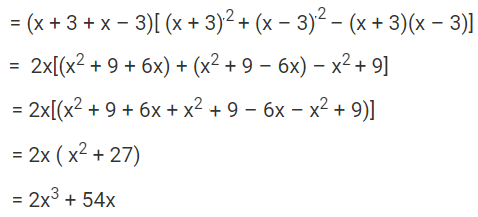(ii) (x/2 + y/3) 3 – (x/2 – y/3) 3

Here a = (x/2 + y/3) and b = (x/2 – y/3)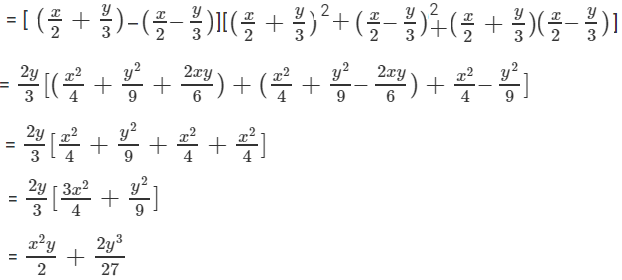(iii) (x + 2/x) 3 + (x – 2/x) 3

Here a = (x + 2/x) and b = (x – 2/x)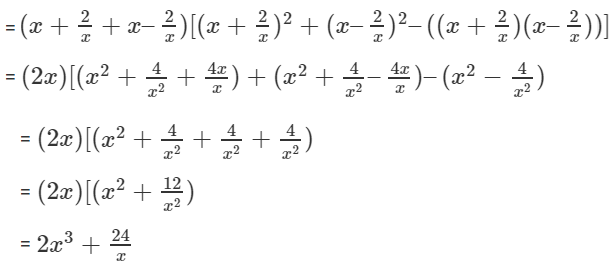(iv) (2x – 5y) 3 – (2x + 5y) 3

Here a = (2x – 5y) and b = 2x + 5y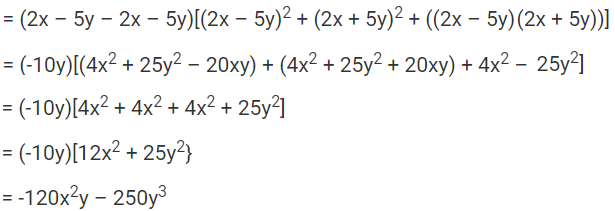Question 3: If a + b = 10 and ab = 21, find the value of a3 + b3.

Solution:

a + b = 10, ab = 21 (given)

Choose a + b = 10

Cubing both sides,

(a + b)3 = (10)3

a3 + b3 + 3ab(a + b) = 1000

a3 + b3 + 3 x 21 x 10 = 1000 (using given values)

a3 + b3 + 630 = 1000

a3 + b3 = 1000 – 630 = 370

or a3 + b3 = 370

Question 4: If a – b = 4 and ab = 21, find the value of a3 – b3.

Solution:

a – b = 4, ab= 21 (given)

Choose a – b = 4

Cubing both sides,

(a – b)3 = (4)3

a3 – b3 – 3ab (a – b) = 64

a3 – b3 – 3 × 21 x 4 = 64 (using given values)

a3 – b3 – 252 = 64

a3 – b3 = 64 + 252

= 316

Or a3 – b3 = 316

Question 5: If x + 1/x = 5, find the value of x3 + 1/x3 .

Solution:

Given: x + 1/x = 5

Apply Cube on x + 1/x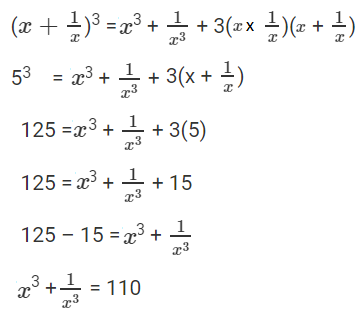Question 6: If x – 1/x = 7, find the value of x3 – 1/x3 .

Solution:

Given: x – 1/x = 7

Apply Cube on x – 1/x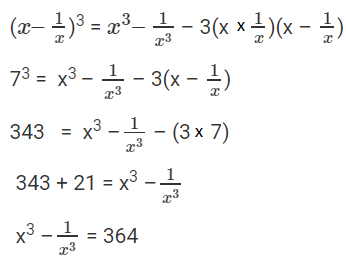Question 7: If x – 1/x = 5, find the value of x3 – 1/x3 .

Solution:

Given: x – 1/x = 5

Apply Cube on x – 1/x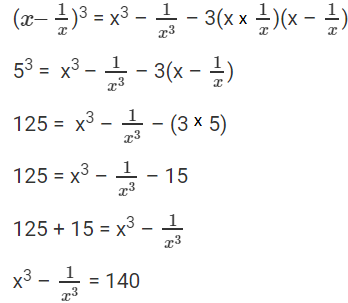Question 8: If (x2 + 1/x2) = 51, find the value of x3 – 1/x3.

Solution:

We know that: (x – y)2 = x2 + y2 – 2xy

Replace y with 1/x, we get

(x – 1/x)2 = x2 + 1/x2 – 2

Since (x2 + 1/x2) = 51 (given)

(x – 1/x)2 = 51 – 2 = 49

or (x – 1/x) = ±7

Now, Find x3 – 1/x3

We know that, x3 – y3 = (x – y)(x2 + y2 + xy)

Replace y with 1/x, we get

x3 – 1/x3 = (x – 1/x)(x2 + 1/x2 + 1)

Use (x – 1/x) = 7 and (x2 + 1/x2) = 51

x3 – 1/x3 = 7 x 52 = 364

x3 – 1/x3 = 364

Question 9: If (x2 + 1/x2) = 98, find the value of x3 + 1/x3.

Solution:

We know that: (x + y)2 = x2 + y2 + 2xy

Replace y with 1/x, we get

(x + 1/x)2 = x2 + 1/x2 + 2

Since (x2 + 1/x2) = 98 (given)

(x + 1/x)2 = 98 + 2 = 100

or (x + 1/x) = ±10

Now, Find x3 + 1/x3

We know that, x3 + y3 = (x + y)(x2 + y2 – xy)

Replace y with 1/x, we get

x3 + 1/x3 = (x + 1/x)(x2 + 1/x2 – 1)

Use (x + 1/x) = 10 and (x2 + 1/x2) = 98

x3 + 1/x3 = 10 x 97 = 970

x3 + 1/x3 = 970

Question 10: If 2x + 3y = 13 and xy = 6, find the value of 8x3 + 27y3.

Solution:

Given: 2x + 3y = 13, xy = 6

Cubing 2x + 3y = 13 both sides, we get

(2x + 3y)3 = (13)3

(2x)3 + (3y) 3 + 3( 2x )(3y) (2x + 3y) = 2197

8x3 + 27y3 + 18xy(2x + 3y) = 2197

8x3 + 27y3 + 18 x 6 x 13 = 2197

8x3 + 27y3 + 1404 = 2197

8x3 + 27y3 = 2197 – 1404 = 793

8x3 + 27y3 = 793

Question 11: If 3x – 2y= 11 and xy = 12, find the value of 27x3 – 8y3.

Solution:

Given: 3x – 2y = 11 and xy = 12

Cubing 3x – 2y = 11 both sides, we get

(3x – 2y)3 = (11)3

(3x)3 – (2y)3 – 3 ( 3x)( 2y) (3x – 2y) =1331

27x3 – 8y3 – 18xy(3x -2y) =1331

27x3 – 8y3 – 18 x 12 x 11 = 1331

27x3 – 8y3 – 2376 = 1331

27x3 – 8y3 = 1331 + 2376 = 3707

27x3 – 8y3 = 3707

## RD Sharma Solutions for Class 9 Maths Chapter 4 Algebraic Identities Exercise 4.3

RD Sharma Solutions Class 9 Maths Chapter 4 Algebraic Identities exercise 4.3 is based on identity for the cube of a binomial.

Important properties:

• (a+b)3 = a3 + b3 + 3ab(a+b)
• (a – b)3 = a3 – b3 – 3ab(a – b)